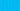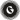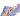PricingResources

# How to Calculate Net Present Value (NPV)Written by

When it comes to investment appraisal, it can be highly beneficial to know how to calculate net present value. Find out exactly what you can learn from net present value and get the lowdown on the best net present value formulas to use for your business.

## .css-g8fzsc{padding:0;margin:0;font-weight:700;}What is Net Present Value (NPV)?

Net present value is the difference between the present value of your cash inflows and the present value of your cash outflows over a given period.

It is used in investment planning and capital budgeting to measure the profitability of projects or investments, similar to accounting rate of return (ARR). Essentially, by looking at all the money you expect to make from an investment and translating those returns into the dollar value of the present day, you can work out whether a particular investment is worthwhile.

The key benefit of NPV is the fact that it considers the time value of money (TVM), translating future cash flows into the value of today’s dollars. Because inflation can erode buying power, NPV provides a much more useful measure of your project’s potential profitability. In addition, net present value formulas provide a single, clear number that managers can compare with the initial investment to work out the success of a project or investment.

## What is the formula for net present value?

Learning how to calculate net present value is relatively straightforward, although it’s important to remember that the formula may vary depending on the consistency and number of cash flows that you’re dealing with.

If the project only has one cash flow, you can use the following net present value formula to calculate NPV:

In this case, i = required return or discount rate and t = number of time periods.

If you’re dealing with a longer project that involves multiple cash flows, there’s a slightly different net present value formula you’ll need to use. However, that’s all relatively abstract, so if you want a simple way to think about net present value formulas, just consider the following:

If you end up with a positive net present value, it indicates that the projected earnings exceed your anticipated costs, and the investment is likely to be profitable. On the other hand, an investment that results in a negative NPV is likely to result in a loss. So, if you’re trying to work out whether to go ahead with an investment, it’s generally a good idea to only focus on projects that offer a positive NPV.

Of course, there’s no reason to calculate NPV by yourself, especially since there are so many NPV calculators available to use instead. Investopedia provides a simple NPV calculator that you can use to determine the difference between the value of your cash inflows and cash outflows.

## ROI vs NPV

Return on investment (ROI) and net present value (NPV) are both methods of evaluating the potential profitability of an investment. But what’s the difference between them? ROI represents the net value you’ll receive from an investment over a given period. The formula for ROI is as follows:

It’s very simple and easy to use, but because it’s so simple, it’s possible that ROI doesn’t tell the whole story. Most importantly, ROI doesn’t consider the time value of money, which makes it a slightly less effective form of measurement, because “future money” may be less valuable than “present money.”

When it comes to ROI vs NPV, it’s important to remember that NPV is a much more complex equation. It pays much closer attention to when the costs and benefits occur before converting them into today’s values. As NPV considers the time value of money, it provides a deeper insight into the viability of your investment options.

## We can help

GoCardless helps you automate payment collection, cutting down on the amount of admin your team needs to deal with when chasing invoices. Find out how GoCardless can help you with ad hoc payments or recurring payments.

Found this article interesting? Take a look at how to calculate Value at Risk (VaR)Contact sales

Sales

Support